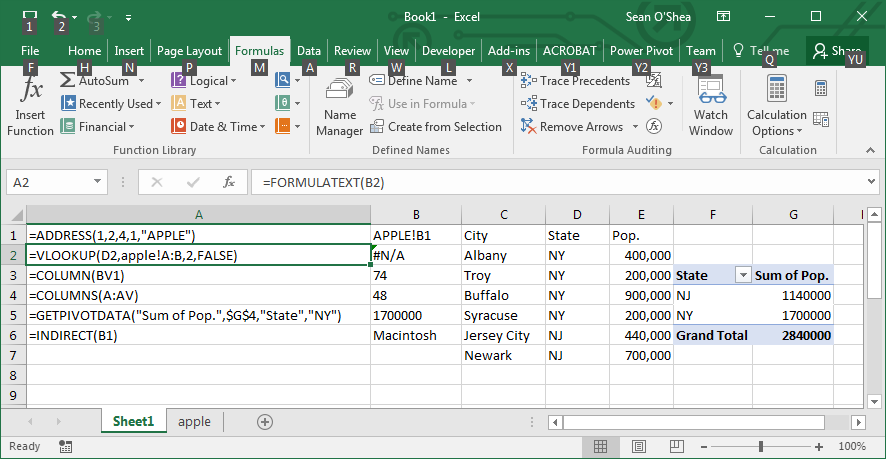Search

# Looking up in Excel

This evening, I'm just running through some basic lookup formulas in Excel , so you can reference things more easily on worksheets when analyzing data.

In column A I have entered the FORMULATEXT formula to show how formulas are entered in column B get the results visible in column B. See the example of the FORMULATEXT shown in the formula bar which reveals the VLOOKUP formula entered in cell B2.

The ADDRESS formula shown in cell A1, gives us in B1 the cell reference that is specified this way:

=ADDRESS(ROW NUMBER, COLUMN NUMBER,ABSOLUTE/RELATIVEREFERENCE (1-4),1,WORKSHEET NAME)

The COLUMN formula shown in cell A3, gives us in B3, the number of the specified column.

The COLUMNS formula shown in cell A4, gives us in B4 the number of columns in a given array. Useful when you're creating VLOOKUP formulas.

The GETPIVOTDATA formula shown in cell A5, gives us in B5 a specific value in the pivot table shown on the right.

=GETPIVOTDATA("Field to Pull Data From",Cell Reference to column containing data to be pulled,Name of Corresponding field, value in the Corresponding Field).

The INDIRECT formula gets you the value for data from a cell referred to in a different cell. In this example, we get the value for cell B1 of the Apple worksheet which is referred to in cell B1 of Sheet1.